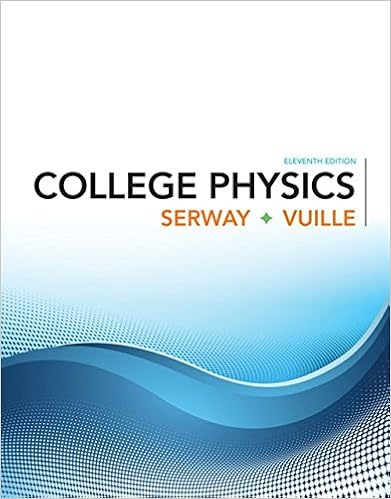# Notice that if we are looking for tangent lines at

• 54
• 100% (2) 2 out of 2 people found this document helpful

This preview shows page 25 - 28 out of 54 pages.

##### We have textbook solutions for you!
The document you are viewing contains questions related to this textbook.The document you are viewing contains questions related to this textbook.
Chapter 1 / Exercise 1.3
College Physics
Serway/VuilleExpert Verified
Notice that if we are looking for tangent lines at the pole, then and Equation 3 sim- plifies to dr d 0 if dy dx tan r 0 dy d 0 dx d 0 dx d 0 dy d 0 dy dx dy d dx d dr d sin r cos dr d cos r sin 3 y r sin f sin x r cos f cos r f 0 2 cos 2 cos 2 sin sin 2 cos cos O (r,   ¨) (_r,   ¨) O (r,   ¨) (r,   _¨) ¨ (a) (b) (c) FIGURE 14 O (r,   ¨) (r,  π -¨) π ¨ 2 r r 644 |||| CHAPTER 10 PARAMETRIC EQUATIONS AND POLAR COORDINATES
##### We have textbook solutions for you!
The document you are viewing contains questions related to this textbook.The document you are viewing contains questions related to this textbook.
Chapter 1 / Exercise 1.3
College Physics
Serway/VuilleExpert Verified
For instance, in Example 8 we found that when or . This means that the lines and (or and ) are tangent lines to at the origin. EXAMPLE 9 (a) For the cardioid of Example 7, find the slope of the tangent line when . (b) Find the points on the cardioid where the tangent line is horizontal or vertical. SOLUTION Using Equation 3 with , we have (a) The slope of the tangent at the point where is (b) Observe that Therefore there are horizontal tangents at the points , , and vertical tangents at and . When , both and are 0, so we must be careful. Using l’Hospital’s Rule, we have By symmetry, Thus there is a vertical tangent line at the pole (see Figure 15). M lim l 3 2 dy dx 1 3 lim l 3 2 sin cos 1 3 lim l 3 2 cos 1 sin lim l 3 2 dy dx lim l 3 2 1 2 sin 1 2 sin lim l 3 2 cos 1 sin dx d dy d 3 2 ( 3 2 , 5 6 ) ( 3 2 , 6 ) ( 1 2 , 11 6 ) ( 1 2 , 7 6 ) 2, 2 when 3 2 , 6 , 5 6 dx d 1 sin 1 2 sin 0 when 2 , 3 2 , 7 6 , 11 6 dy d cos 1 2 sin 0 1 s 3 1 s 3 1 1 s 3 ( 2 s 3 )( 1 s 3 ) 1 2 ( 1 s 3 ) ( 1 s 3 2 )( 1 s 3 ) dy dx 3 cos 3 1 2 sin 3 1 sin 3 1 2 sin 3 3 cos 1 2 sin 1 2 sin 2 sin cos 1 2 sin 1 sin 1 2 sin dy dx dr d sin r cos dr d cos r sin cos sin 1 sin cos cos cos 1 sin sin r 1 sin 3 r 1 sin r cos 2 y x y x 3 4 4 3 4 4 r cos 2 0 SECTION 10.3 POLAR COORDINATES |||| 645 5 π 6      ,         3 2 7 π 6      ,         1 2      ,          11 π 6 1 2 3 2 π 6     ,      (0,   0) m=_1 ”1+        ,      π 3 oe „3 2 ”2,       π 2 FIGURE 15 Tangent lines for r=1+ sin   ¨
Instead of having to remember Equation 3, we could employ the method used to derive it. For instance, in Example 9 we could have written Then we would have which is equivalent to our previous expression. GRAPHING POLAR CURVES WITH GRAPHING DEVICES Although it’s useful to be able to sketch simple polar curves by hand, we need to use a graphing calculator or computer when we are faced with a curve as complicated as the ones shown in Figures 16 and 17. Some graphing devices have commands that enable us to graph polar curves directly. With other machines we need to convert to parametric equations first. In this case we take the polar equation and write its parametric equations as Some machines require that the parameter be called rather than . EXAMPLE 10 Graph the curve . SOLUTION Let’s assume that our graphing device doesn’t have a built-in polar graphing command. In this case we need to work with the corresponding parametric equations, which are In any case, we need to determine the domain for . So we ask ourselves: How many complete rotations are required until the curve starts to repeat itself? If the answer is , then sin 8 2 n 5 sin 8 5 16 n 5
•••